SYLLABUS  Previous: 1.5.1 Drift and volatility  Up: 1.5 Historical data and  Next: 1.5.3 Maximum likelihood estimate

1.5.2 Moving averages: UWMA, EWMA, GARCH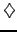[ SLIDE UWMA - EWMA - GARCH || VIDEO modem - LAN - DSL ]

Consider a sequence of spot prices {S1, S2, ...}   obtained from the market at regular time intervals labelled i=1,2,3,... Introduce the normalized increments si=ln(Si/Si-1)   which, it will be shown in the next chapter, are typical of a log-normal distribution of the price increments observed on the stock market. Following Markowitz' definition of volatility as standard deviation of the expected return, it is useful first to estimate the mean (drift) and the variance (square of volatility) per unit time Dt using the m   most recent observations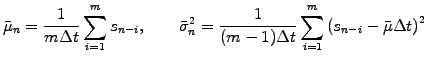(1.5.2#eq.1)

This formula provides the basis for the so-called uniformly weighted moving avergage (UWMA) and has been implemented in the MKTSolution applet,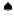using a 6 months window for the volatility and a 5 years window for the drift-there are 252 trading days during a year. The drift and the volatility is finally expressed on an annual basis with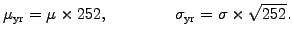(1.5.2#eq.2)

For small changes ln(Si/Si-1) = ln((Si-1+dSi/Si-1) = ln(1+ dSi/Si-1) » dSi/Si-1   the normalized increments are generally approximated with the ratio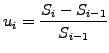(1.5.2#eq.3)

and the small drift associated with the mean is generally neglected in comparison to the much larger fluctuating component. For a large number m»m-1   the formula for the variance can then be simplified to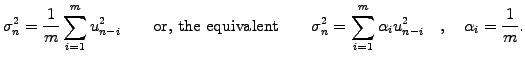(1.5.2#eq.4)

The UWMA of drift, volatility and other quantities that can be estimated from time series, such as correlations, suffers from two main short-commings: the most recent events that are most significant, only carry the same uniform weight ai   as all the others in the averaging window, this until the information is abruptly lost after m days. In addition, the UWMA is independent of a long term average towards which temporary deviations tend to revert to.
To tackle the first problem, the average window can be dropped in favour of a recursive or auto-regressive definition, producing an exponentially weighted moving average (EWMA) where the last known quantity is constantly updated with the most recent market increment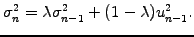(1.5.2#eq.5)

Insert (1.5.2#eq.5) back in itself and work through the recursion a few times to convince yourself that the weights, which were uniform in (1.5.2#eq.4), now are exponentially decaying with a forgetting rate'' ai= (1-l)/li-1 that accelerates as l Î [0;1]   gets smaller (exercise 1.09). In its RiskMetrics database, J.P.Morgan for example uses an EWMA model with l=0.94,   and has also been implemented in the MKTSolutionapplet. Alternatively, a maximum likelyhood estimate can be calculated for every spot price using the method described in the next section (exercise 1.05).
The second issue is generally solved by writing the long term average as V=w/(1-a-b)   and introducing a reversion term in a so-called generalized auto-regressive conditional heteroscedasticity model, using the p most recent increments and the q most recent volatility estimates in GARCH(p,q). The most commonly used is GARCH(1,1)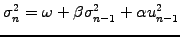(1.5.2#eq.6)

where a   controls the sensitivity to most recent increments, b   the forgetting rate and w=gV   is linked with long term average. For consistency, the parameters must satisfy a+b+g=1   and to prevent negative long term average volatility, it is important that a+b<1.   Clearly, the EWMA model is a particular case of GARCH(1,1), where w=0, a=1-l, b=l.
MKTSolution applet:  select one or several of the uniform UWMA, exponential EWMA or GARCH(1,1) averaging models to calculate the volatility of the General Motors share and press Draw to plot them as a function of the trading days during the year 2001. You can perform measurements by clicking inside the plot area and access up-to-date market data for a broad range of symbols under the previous link.

 Market data: volatility models Plot the price history and the market volume for shares in General Motors during one trading year and examine how they affect the volatility. Compare different volatility models and try to summarize their response to a market event such as the WTC attack on Sep 11, 2001. Follow the link to the Market solution applet and compare the model volatilities with the values quoted elsewhere on the Internet.

Qualitative arguments support models with features such as the exponential weighting (forgetting''), a reversion mechanism (long term average'') and the tendency to reproduce the auto-correlation of the market (clustering'', i.e. large ui2 tends to produce large ui+12, ui+22, etc   ). Since the volatility is not a quantity that can be directly measured on the market, it is not easy to judge which model is better or worse. Nevertheless, an independent test could compare the implied volatility of options (later defined in sect.4.1.3) with the value calculated here using the underlying share. The plot in (1.5.1#fig.1,bottom) shows such a comparison for Cisco during the period 2001-2004: the EWMA calculated from the stock market history assuming the parameter l=0.94   does indeed accurately reproduce the implied volatility calculated from the option market, except in 2001 during the period of high volatility when the EWMA appears to predict larger values. Apart from following the advice from financial institutions, is there an independent way to calibrate the parameters a, b, l, w,   in a manner that achieves the best possible fit between a model and the data? Yes, this will be the last topic of this introduction.

SYLLABUS  Previous: 1.5.1 Drift and volatility  Up: 1.5 Historical data and  Next: 1.5.3 Maximum likelihood estimate